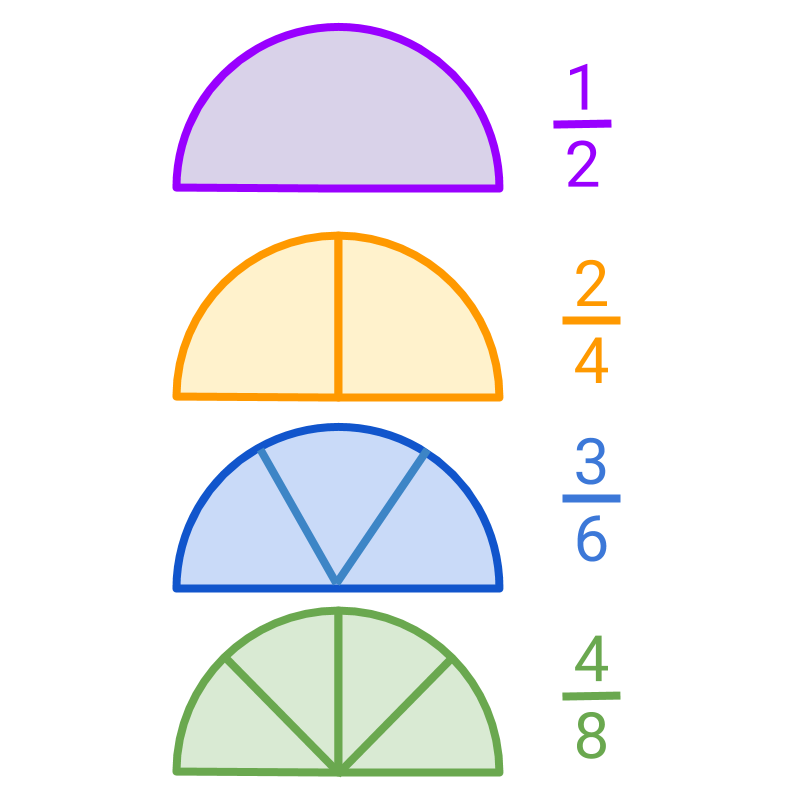maths > fractions

Simplest form of a fraction

what you'll learn...

overview

In this, finding the simplest form of a fraction is explained.

•  The numerator and denominator are divided by any common factors to find the simplest from of the fraction.

simple among the equalsThe picture shows four fractions , $\frac{1}{2}$$\frac{1}{2}$, $\frac{2}{4}$$\frac{2}{4}$, $\frac{3}{6}$$\frac{3}{6}$, and $\frac{4}{8}$$\frac{4}{8}$. These are equivalent fractions.

A fraction without a common factor between the numerator and the denominator is the simplest form of the equivalent fractions.

The numerator and denominator are divided by any common factors to find the simplest from of the fraction.

A fraction without a common factor between the numerator and the denominator is the simplest form of the equivalent fractions.

Finding Simplest form of a fraction: Given a fraction $\frac{p}{q}$$\frac{p}{q}$, to find the simplest form of the fraction
find the highest common factor $i$$i$ such that $p=l×i$$p = l \times i$ and $q=m×i$$q = m \times i$. Then the simplest form is $\frac{l}{m}$$\frac{l}{m}$."

What is the simplest form of $\frac{35}{45}$$\frac{35}{45}$?
The answer is '$\frac{7}{9}$$\frac{7}{9}$'. The HCF of numerator and denominator is $5$$5$ which is canceled in both numerator and denominator. $\frac{35}{45}=\frac{7×5}{9×5}=\frac{7}{9}$$\frac{35}{45} = \frac{7 \times 5}{9 \times 5} = \frac{7}{9}$

summary

»  Cancel any common factors of numerator and denominatoreg: Simplest form of $\frac{2}{4}$$\frac{2}{4}$, $\frac{3}{6}$$\frac{3}{6}$, and $\frac{4}{8}$$\frac{4}{8}$ is $\frac{1}{2}$$\frac{1}{2}$.

Outline